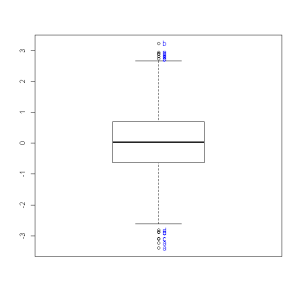## How to label all the outliers in a boxplot

In this post I offer an alternative function for boxplot, which will enable you to label outlier observations while handling complex uses of boxplot.

In this post I present a function that helps to label outlier observations When plotting a boxplot using R.

An outlier is an observation that is numerically distant from the rest of the data. When reviewing a boxplot, an outlier is defined as a data point that is located outside the fences (“whiskers”) of the boxplot (e.g: outside 1.5 times the interquartile range above the upper quartile and bellow the lower quartile).

Identifying these points in R is very simply when dealing with only one boxplot and a few outliers. That can easily be done using the “identify” function in R. For example, running the code bellow will plot a boxplot of a hundred observation sampled from a normal distribution, and will then enable you to pick the outlier point and have it’s label (in this case, that number id) plotted beside the point:

```set.seed(482)
y <- rnorm(100)
boxplot(y)
identify(rep(1, length(y)), y, labels = seq_along(y))
```

However, this solution is not scalable when dealing with:

• Many outliers
• Overlapping data-points, and
• Multiple boxplots in the same graphic window

For such cases I recently wrote the function "boxplot.with.outlier.label" (which you can download from here). This function will plot operates in a similar way as "boxplot" (formula) does, with the added option of defining "label_name". When outliers are presented, the function will then progress to mark all the outliers using the label_name variable. This function can handle interaction terms and will also try to space the labels so that they won't overlap (my thanks goes to Greg Snow for his function "spread.labs" from the {TeachingDemos} package, and helpful comments in the R-help mailing list).

Here is some example code you can try out for yourself:

```source("https://raw.githubusercontent.com/talgalili/R-code-snippets/master/boxplot.with.outlier.label.r") # Load the function
# sample some points and labels for us:
set.seed(492)
y <- rnorm(2000)
x1 <- sample(letters[1:2], 2000,T)
x2 <- sample(letters[1:2], 2000,T)
lab_y <- sample(letters[1:4], 2000,T)
# plot a boxplot with interactions:
boxplot.with.outlier.label(y~x2*x1, lab_y)
```

Here is the resulting graph:You can also have a try and run the following code to see how it handles simpler cases:

```# plot a boxplot without interactions:
boxplot.with.outlier.label(y~x1, lab_y, ylim = c(-5,5))
# plot a boxplot of y only
boxplot.with.outlier.label(y, lab_y, ylim = c(-5,5))
boxplot.with.outlier.label(y, lab_y, spread_text = F) # here the labels will overlap (because I turned spread_text off)
```

Here is the output of the last example, showing how the plot looks when we allow for the text to overlap (we would often prefer to NOT allow it).Regarding package dependencies: notice that this function requires you to first install the packages {TeachingDemos} (by Greg Snow) and {plyr} (by Hadley Wickham)